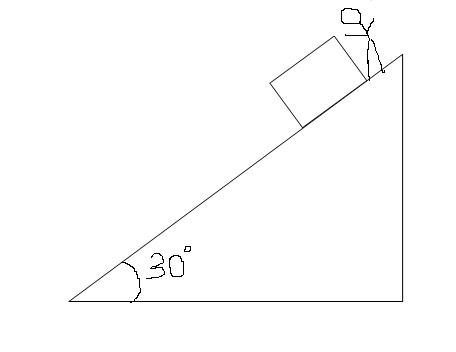# QuestionsQ1) A man wants to slide down a block of mass $m$ which is kept on a fixed inclined plane of inclination $30^{o}$ as shown . Initially, the block is not sliding. to just start sliding, the man pushes the block down the incline with a force $F$. Now the block starts accelerating. To move it downwards with constant speed, the man starts pulling the block with the same force. Surfaces are such that ratio of maximum static friction to kinetic friction is $2$.

a)what is the value of $F$

b) If the man wants to move the block up the incline, what is minimum force required to just start the motion

c) What is the minimum force required to move it up the incline with constant speed

d) If the man continues pushing the block by force by force $F$, the acceleration would beNote by Tanishq Varshney
6 years, 2 months ago

This discussion board is a place to discuss our Daily Challenges and the math and science related to those challenges. Explanations are more than just a solution — they should explain the steps and thinking strategies that you used to obtain the solution. Comments should further the discussion of math and science.

When posting on Brilliant:

• Use the emojis to react to an explanation, whether you're congratulating a job well done , or just really confused .
• Ask specific questions about the challenge or the steps in somebody's explanation. Well-posed questions can add a lot to the discussion, but posting "I don't understand!" doesn't help anyone.
• Try to contribute something new to the discussion, whether it is an extension, generalization or other idea related to the challenge.

MarkdownAppears as
*italics* or _italics_ italics
**bold** or __bold__ bold
- bulleted- list
• bulleted
• list
1. numbered2. list
1. numbered
2. list
Note: you must add a full line of space before and after lists for them to show up correctly
paragraph 1paragraph 2

paragraph 1

paragraph 2

[example link](https://brilliant.org)example link
> This is a quote
This is a quote
    # I indented these lines
# 4 spaces, and now they show
# up as a code block.

print "hello world"
# I indented these lines
# 4 spaces, and now they show
# up as a code block.

print "hello world"
MathAppears as
Remember to wrap math in $$ ... $$ or $ ... $ to ensure proper formatting.
2 \times 3 $2 \times 3$
2^{34} $2^{34}$
a_{i-1} $a_{i-1}$
\frac{2}{3} $\frac{2}{3}$
\sqrt{2} $\sqrt{2}$
\sum_{i=1}^3 $\sum_{i=1}^3$
\sin \theta $\sin \theta$
\boxed{123} $\boxed{123}$

Sort by:6#8&7#

- 6 years, 2 months ago

thanks @Kushal Patankar @Nishant Rai for the help

- 6 years, 2 months ago

- 6 years, 2 months ago

Can you guys help me here?

- 6 years, 2 months ago

Tanishq I need your help here

- 6 years, 2 months ago

Let coefficient of friction be $\eta_s , \eta_k$ then $\frac{\eta_s N}{\eta_k N}=2 \rightarrow$ $\frac{\eta_s}{\eta_k} = 2$

for the block to just move downward, forces should balance each other along the incline.

i am just writing the equations, check if these are correct.

$F+ mg \sin \theta = \eta_s mg \cos \theta ......(i)$ in case of just sliding downwards.

$F= mg \sin \theta - \eta_k mg \cos \theta .....(ii)$ in case of pulling it upwards.

equating the first two equations, we get $\eta_k = \frac{2}{3\sqrt{3}}$

On substituting the value of $\eta_k$ in eq $(ii)$, we get $\frac{mg}{6}$

- 6 years, 2 months ago

$b)$ $F - mg \sin \theta - \eta_s mg \cos \theta =0$

$c)$ $F - mg \sin \theta - \eta_k mg \cos \theta =0$

$d)$ $ma = F + mg \sin \theta - \eta_k mg \cos \theta \rightarrow a = \frac{g}{6}$

- 6 years, 2 months ago

@Nishant Rai @Saurabh Patil @satvik pandey @Kushal Patankar plz help by posting solution

- 6 years, 2 months ago

Looks I am late. :) There was some problem with internet connection that's why I was unable to help you.

- 6 years, 2 months ago

no problem, the above problem was sorted out

- 6 years, 2 months ago

How is preparations going on, for ADVANCE?

- 6 years, 2 months ago

- 6 years, 2 months ago

- 6 years, 2 months ago

$\frac{mg}{6}$

- 6 years, 2 months ago

answers to $b,c,d$ ?

- 6 years, 2 months ago

b) 7mg/6

c) 5mg/6

d) g/3

- 6 years, 2 months ago

- 6 years, 2 months ago

i have a doubt. On solving the equations, we get $\eta_s = \frac{4}{3\sqrt{3}}$ which is greater than $\tan \theta$ , which means the body will not move on its own down the inclined plane. Then how in the second case, the body starts accelerating downwards , and a pulling force is required to move the block with a constant speed? i mean the body cannot move on its own if $\tan \theta < \eta$ .

- 6 years, 2 months ago

Do you think static friction will act at its maximum value.

- 6 years, 2 months ago